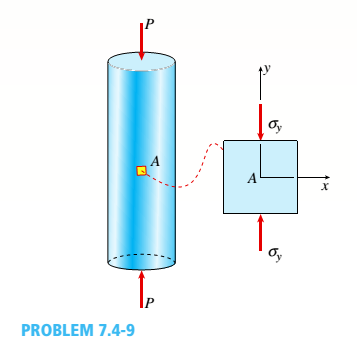# A specimen used in a coupon test is shown in the figure. The stresses on element A are known to be s y = -1500 psi. Use Mohr’s circle to: (a) Find the stresses acting on the element oriented at an angle ?? = -35°. (b) Find maximum normal and shear stresses and show them on sketches of properly oriented elements.### Mechanics of Materials (MindTap Co...

9th Edition
Barry J. Goodno + 1 other
Publisher: Cengage Learning
ISBN: 9781337093347
Chapter 7, Problem 7.4.9P
Textbook Problem

## A specimen used in a coupon test is shown in the figure. The stresses on element A are known to be sy= -1500 psi. Use Mohr’s circle to: (a) Find the stresses acting on the element oriented at an angle ?? = -35°. (b) Find maximum normal and shear stresses and show them on sketches of properly oriented elements.Expert Solution

(a)

To determine

The state of stress on the element oriented at angle 35°.

### Explanation of Solution

Given information:

The normal stress at point A along y-axis is 1500psiand orientation of element is 35°.

Write the expression for average stress.

σavg=σx+σy2(I)

Here, average stress is σavg, normal stress along x-direction is σx, and normal stress along y-direction is σy.

Write the expression for the radius of Mohrs circle.

R=(σx+σy2)2+τxy(II)

Here, the radius of the Mohrs circle is Rand shear stress along xy-plane is τxy.

Write the expression for the normal stress on element along x-direction.

σx1=σavg+Rcos(2θ)(III)

Here, normal stress on element along x-direction is σx1and orientation of the element is θ.

Write the expression for the normal stress on element along y-direction.

σy1=σavgRcos(2θ)(IV)

Here, normal stress on element along y-direction is σy1.

Write the expression for shear stress on the element.

τx1y1=Rsin(2θ)(V)

Here, shear stress on oriented element is τx1y1.

Calculation:

Substitute 0for σxand 1500psifor σyin equation (I).

σavg=0psi+(1500)psi2=1500psi2=750psi

Substitute 0for σx, 1500psifor σy, and 0for τxyin equation (II).

R=(0psi+(1500psi)2)2+(0psi)2=(750psi)2=750psi

Substitute 750psifor σavg, 750psifor Rand 35°for θin equation (III)

### Want to see the full answer?

Check out a sample textbook solution.See solution

### Want to see this answer and more?

Bartleby provides explanations to thousands of textbook problems written by our experts, many with advanced degrees!

See solution